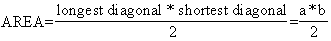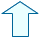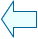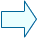Geometric shapes on the Plane_3 Section: Geometry

 1. THE AREA OF A RHOMBUS (1st WAY) The rhombus is a special kind of parallelogram with four equal sides. If we know the base and height we can use the formula for working out the area of a parallelogram. However, the area of a rhombus is often expressed by referring to its diagonal lines. The area of a rhombus, in terms of its diagonals is:In the shape below you can see that we have called the diagonals a and b. We have also drawn a rectangle around the rhombus as we are going to use the rectangle to help us work out the area of a rhombus. 1.- Click on the button and four green triangles should appear which are the same as the four triangles that make up the rhombus. 2.- Therefore we can say that the area of a rhombus is half of the area of a rectangle.

 2. THE AREA OF A  RHOMBUS ( 2nd WAY ) In the following window we can see how a different construction can be used to work out the area of a rhombus. 1.- Follow all the steps indicated by the window and then try to explain how this demonstration works to find out the area. 2.- Go back to the area of a triangle page and review the window where we constructed different triangles with the same area. This will help you to understand step 3.Agustín Muñoz NúñezSpanish Ministry of Education. Year 2001